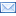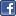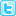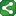Friday, November 21, 2008

How to detect missing values using ARRAYS

Using an array in SAS to detect missing values

Question:
How do I exclude observations from my PROC FREQ analysis when a value is missing from a list of variables?

In the SAS DATA step, you can create a new variable ("miss" in the example below) that is set equal to 1 when a variable has a missing value, 0 otherwise. Use the ARRAY statement and a DO loop to check for missing values across a list of variables; the syntax is:

DATA one ;
INFILE xxx;
INPUT a b c d e;
miss=0;
ARRAY vv(5) a b c d e ;
DO i=1 TO 5 ;
IF vv(i)=. THEN DO;
miss=1 ;
i=5;

END;
END;
RUN;

PROC FREQ;
WHERE miss =0;
TABLES a b c d e ;
RUN ;

Here, the array "vv" has 5 elements (a,b,c,d,e), and the loop "i" is likewise set to 5.

For each observation, the loop iterates 5 times, checking for missing values across the list of 5 variables. When a missing value is encountered, the variable "miss" is set to 1 and the loop stopped for that observation.

"Miss" was initially set to zero, and it is only changed if an observation has missing data on any of the five variables. The PROC FREQ then uses the WHERE statement to restrict processing to observations having "miss" set to zero.
Want to know more about Missing Values...ShareThis